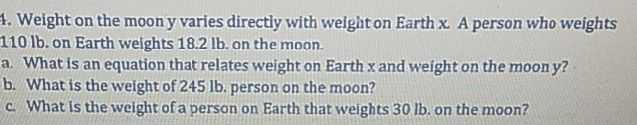### ¿Todavía tienes preguntas de matemáticas?

Pregunte a nuestros tutores expertos
Algebra
Pregunta4. Weight on the moon y varies directly with weight on Earth x. A person who weights

$$110 lb$$ . on Earth weights $$18.2 lb$$ . on the moon. a. What is an equation that relates weight on Earth $$x$$ and weight on the moon $$y$$ ? b. What is the weight of $$245 lb$$ . person on the moon? c. What is the weight of a person on Earth that weights $$30$$ lb. on the moon?